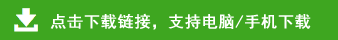# 部编版三年级下册语文综合整理

(崇高)     乏 (缺乏)   减 (减少)   奏 (奏乐）

(宗旨)     泛 (广泛)   喊 (呼喊)   凑 (凑合)

(吹拂)     聚 (聚会)   形 (形状)   掠 (掠过)

(遇到)     站 (站立)   倦（疲倦）  辫（辫子）

(莲蓬)     资 (资本)   仿（仿照）  矩（规矩）

(篷车)     姿 (姿态)   访（访问）  距（距离）

(丝绸)     害 (害怕)   约 (大约)   斑 (斑马)

(稠密)     瞎 (瞎说)   药 (吃药)   班 (班级）

(洗浴)  魂(灵魂)  借(借书)  洒(洒水）

(欲望)  魄(气魄)  错(错误)  酒(喝酒）

(独自)  异(异乡)  佳(佳节)  欧(欧洲）

(烛光)  导(开导)  住(住房)  鸥(海鸥）

(经验)  县(县城)  史(历史)  历(日历）